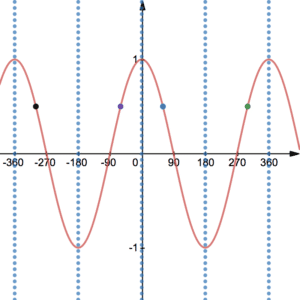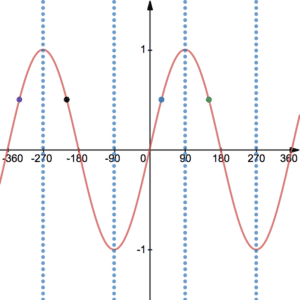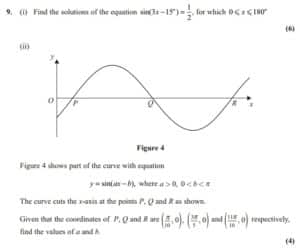# Trigonometric Graphs

We derive the trigonometric graphs of sin, cos and tan from the unit circle. Imagine a particle going round the unit circle. Let $\theta$ measure the anti-clockwise angle between the particle and the $x$-axis. We can think of the value of $\cos(\theta)$ as the $x$ coordinate of the particle. Similarly, we can think of the value of $\sin(\theta)$ the $y$ coordinate. See the table  below.

Exam questions on trigonometric graphs may expect you to have memorised these values. Additionally, you should also know values of sin and cos at 30 and 60 degrees. See Trigonometric Identities. They may also expect you to perform transformations to trigonometric graphs. See Examples 1 and 2 here after studying the graphs below. Click here for a reminder of Transformations.

## The graphs of cos and sin

We now plot the $x$-coordinate of the particle against the angle. Calculating the coordinates for all $\theta$ using a scientific calculator will give the following curve:Local lines of symmetry are vertical lines at every $180^\circ$.
In other words, they have equation $x=180n$ where $n\in{\mathbb Z}$

We can also plot the $y$-coordinate against the angle of the particle. Calculating the coordinates for all $\theta$ using a scientific calculator will give the following curve:Local lines of symmetry are vertical lines every $180^\circ$ starting at $90^\circ$. In other words, they have equation $x=180n-90$ (or equivalent) where $n\in{\mathbb Z}$.

Both $y$ values on the sin and cos graphs are bounded between -1 and 1. The tan graph, however, can take values between $-\infty$ and $\infty$. Both cos and sin graphs repeat every $360^\circ$. We say that they have periodicity of $360^\circ$.
When solving trigonometric equations, scientific calculators tend to only give default solutions. However, the lines of symmetry above can be used to find all solutions in a given interval. See Trigonometric Equations.

For obvious reasons, sin and cos graphs are sometimes called waves. You may also be interested in the amplitude and wavelength. Firstly, amplitude is the height of the wave. Secondly, the wavelength is how long a single wave is.

## The graph of tan

Recall that $\tan(\theta)=\frac{\sin(\theta)}{\cos(\theta)}$ from the trigonometric identities. The graph of $\tan(\theta)$ is given below. It can be thought of as the ratio of vertical to horizontal components of the above particle.

As opposed to cos and sin, tan is not restricted to taking values between -1 and 1. Tan can in fact have any positive or negative value. However, the tan graph has asymptotes – lines that are never touched. These lines occur every $180^\circ$ starting at $90^\circ$. They appear as a result of dividing by $\cos(\theta)=0$ in the expression $\tan(\theta)=\frac{\sin(\theta)}{\cos(\theta)}$.  Tan has no reflectional lines of symmetry but it does have a periodicity of $180^\circ$.

## Examples of Trigonometric Graphs

Sketch the graph of $y=2\cos(\theta)$.

Sketch the graph of $y=\sin(2\theta)$.Note that this question requires knowledge of radians (coming soon). This is an alternative angle measurement to degrees. Specifically, $180^\circ$ is $\pi$ radians. This means that $360^\circ=2\pi$ etc.

You will also need to be very careful with the transformations. Namely, identify the transformations when replacing $x$ with $ax-b$ carefully.  Firstly, replace $x$ with $x-b$. Then replace new $x$ with $a$x. Of course that is if you are choosing to use transformations. An alternative would be to substitute coordinates.

See video below for full solution.